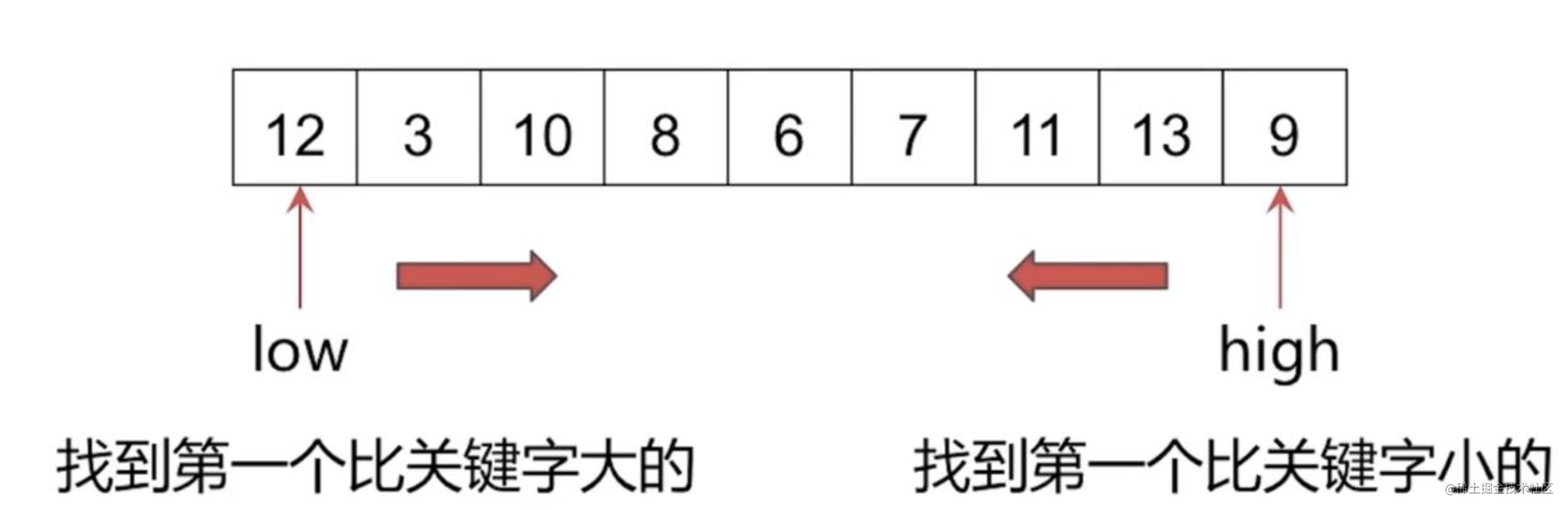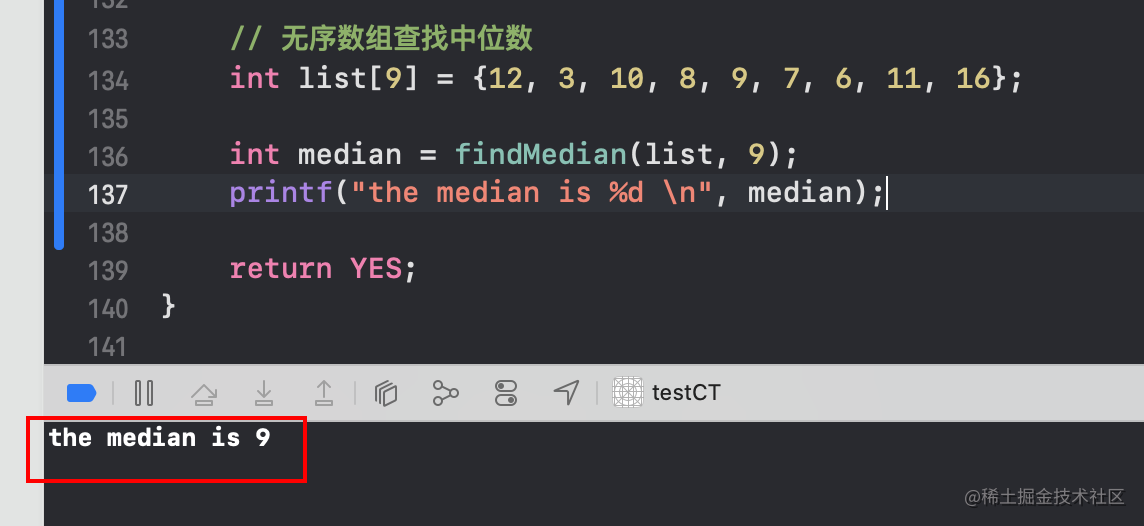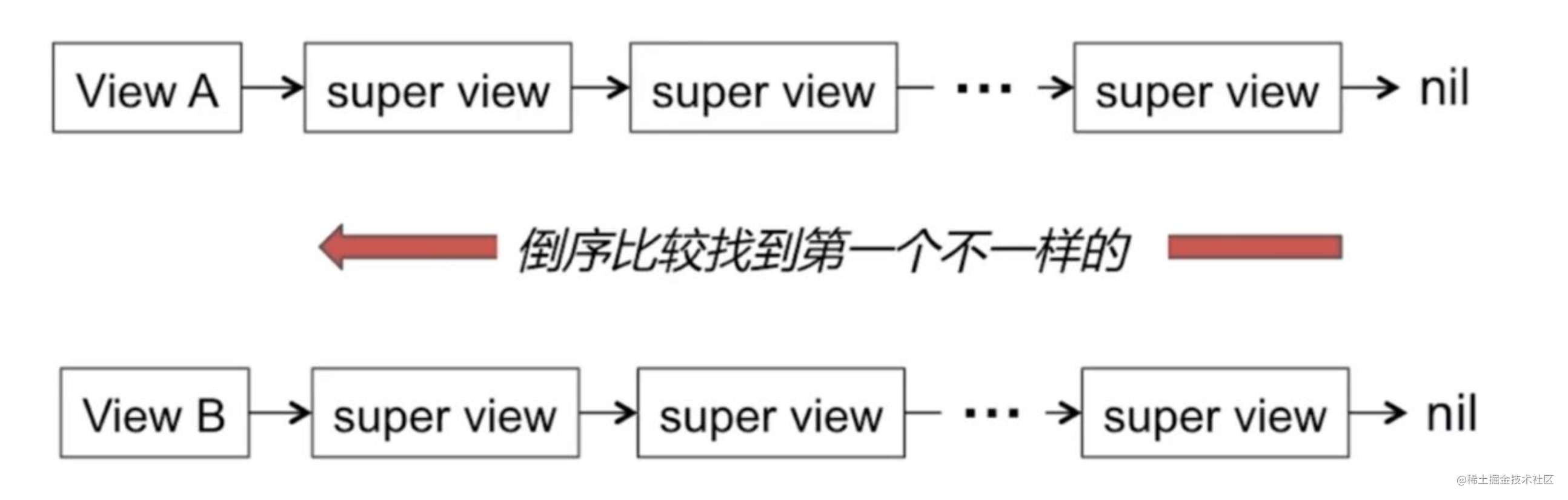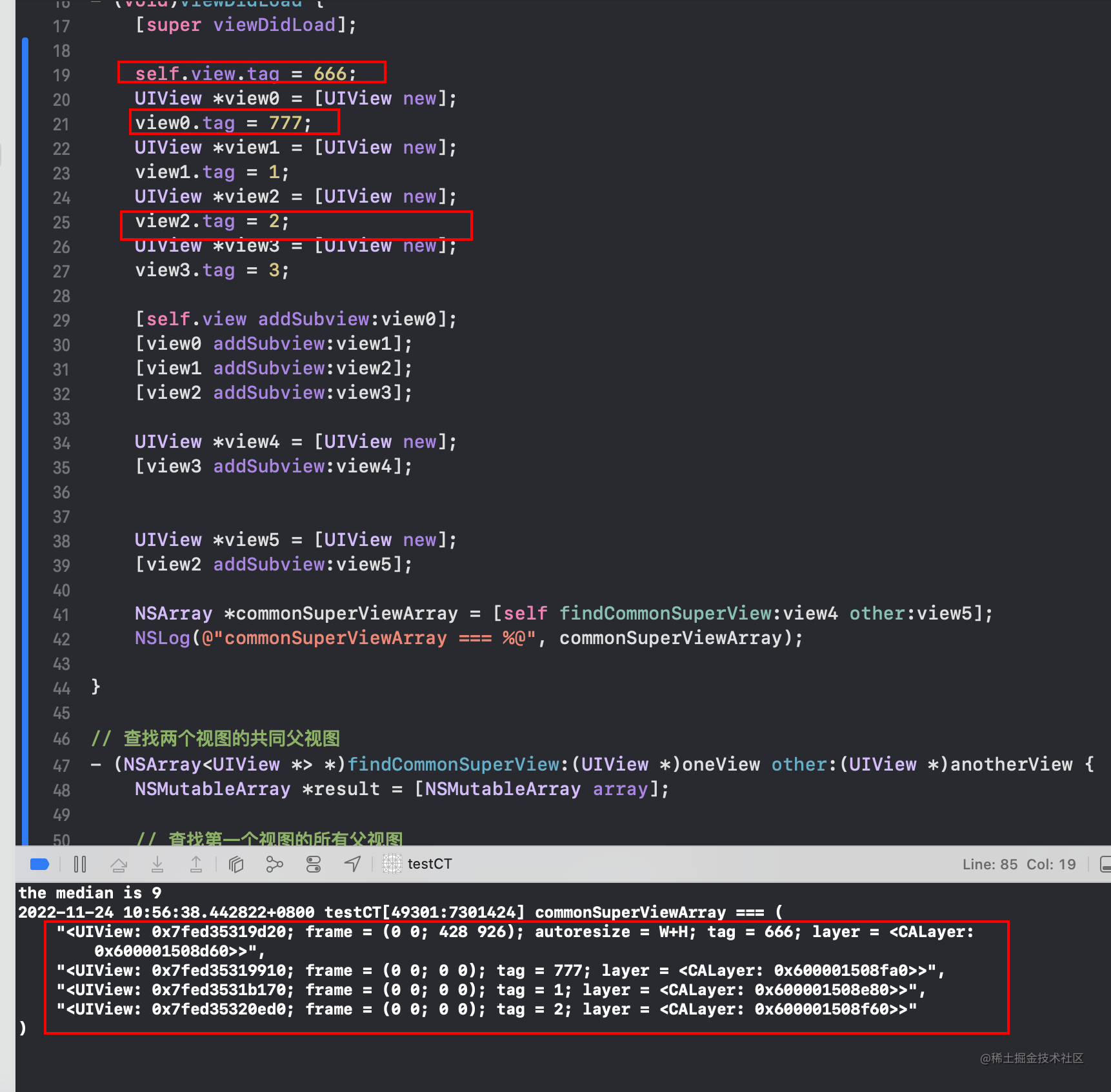# iOS老司機整理, iOSer必會的經典演算法_2

## 前言

• iOS日常開發中, 演算法使用的多嗎? 實事求是的來說, 是不多的.
• 那演算法的學習對iOSer來說, 就不需要了嗎? 答案是很需要.
• iOS的日常開發中, 用到的演算法確實不多, 但是演算法在iOS開發裡面的應用都被封裝在各種常用API的內部了.
• 對演算法的學習, 可以提高對iOS底層的理解與認識, 這些演算法的知識是計算機技術領域通用的. 下面就iOS開發中一些經典的演算法進行一個簡單的梳理.
• 文章純手打, 拋磚引玉, 如有錯誤還請評論區指正, 先行謝過了:)

# 1. 雜湊演算法, 在一個字串中找到第一個, 只出現一次的字元.

• 在字串 s 中找出第一個只出現一次的字元。如果沒有，返回一個單空格。 s 只包含小寫字母。 ``` 輸入：s = "abaccdeff" 輸出：'b'

``````char result = '\0';
// 1. 定義一個數組, 用來儲存各個字母出現的次數
int array;

// 2. 對陣列進行初始化操作
for (int i = 0; i < 256; i++) {
array[i] = 0;
}

// 3. 定義一個指標, 指向當前字串頭部
char *p = s;

// 4. 遍歷每個字元, 在字母對應儲存位置, 進行出現次數+1操作
while (*p != '\0') {
array[*(p++)]++;
}

// 5. 將P指標重新指向字串頭部
p = s;

// 6. 遍歷每個字母出現次數, 遇到第一個出現次數為1的字元, 列印結果, 反之繼續向後遍歷
while (*p != '\0') {
if (array[*p] == 1) {
result = *p;
return result;
break;
}

p++;
}

return ' ';
``````

} ``` - 力扣連結

# 2. 求無序陣列當中的中位數

## 2.1 排序演算法 + 中位數

• 氣泡排序 快速排序 堆排序
• 中位數 ```當n為奇數時, (n + 1)/2 當n為偶數時, (n/2 + (n/2 + 1))/2```

## 2.2 利用快排思想(分治思想)

• 選取關鍵字, 高低指標交替掃描
• 找到第一個比關鍵字大的
• 找到第一個比關鍵字小的1. 任意挑一個元素, 以該元素為支點, 劃分集合為兩部分.
2. 如果左側集合長度恰為`(n-1)/2`, 那麼支點恰為中位數.
3. 如果`左側長度<(n-1)/2`, 那麼中位點在右側; 反之, 中位數在左側.
4. 進入相應的一側繼續尋找中位點.

5. 使用C語言的解答: ``` int findMedian(int a[], int aLen) { int low = 0; int high = aLen -1;

int mid = (aLen - 1) / 2; int div = PartSort(a, low, high);

while (div != mid) { if (mid < div) { // 左半區間找 div = PartSort(a, low, div - 1); } else { // 右半區間找 div = PartSort(a, div + 1, high); } }

// 找到了 return a[mid]; }

int PartSort(int a[], int start, int end) { int low = start; int high = end;

``````// 選取關鍵字
int key = a[end];

while (low < high) {
// 左邊找比key大的值
while (low < high && a[low] <= key) {
++low;
}

// 右邊找比key小的值
while (low < high && a[high] >= key) {
--high;
}

if (low < high) {
// 找到之後交換左右的值
int temp = a[low];
a[low] = a[high];
a[high] = temp;
}
}

int temp = a[high];
a[high] = a[end];
a[end] = temp;

return low;
``````

} ```

• 驗證程式碼: ``` // 無序陣列查詢中位數 int list = {12, 3, 10, 8, 9, 7, 6, 11, 16};

int median = findMedian(list, 9); printf("the median is %d \n", median); ```# 3. 查詢兩個子檢視的共同父檢視

• 演算法思路圖解:• 使用Objective-C語言的解答: ```

self.view.tag = 666;     UIView view0 = [UIView new];     view0.tag = 777;     UIView view1 = [UIView new];     view1.tag = 1;     UIView view2 = [UIView new];     view2.tag = 2;     UIView view3 = [UIView new];     view3.tag = 3;

UIView *view4 = [UIView new];     [view3 addSubview:view4];

UIView *view5 = [UIView new];     [view2 addSubview:view5];

NSArray *commonSuperViewArray = [self findCommonSuperView:view4 other:view5];     NSLog(@"commonSuperViewArray === %@", commonSuperViewArray); }

// 查詢兩個檢視的共同父檢視 - (NSArray )findCommonSuperView:(UIView )oneView other:(UIView )anotherView {     NSMutableArray result = [NSMutableArray array];

// 查詢第一個檢視的所有父檢視     NSArray arrayOne = [self findSuperViews:oneView];     // 查詢第一個檢視的所有父檢視     NSArray arrayOther = [self findSuperViews:anotherView];

int i = 0;     // 越界限制條件     while (i < MIN(arrayOne.count, arrayOther.count)) {         // 倒序方式獲取各個檢視的父檢視         UIView superOne = [arrayOne objectAtIndex:arrayOne.count - i - 1];         UIView superOhter = [arrayOther objectAtIndex:arrayOther.count - i - 1];

// 比較如果相等, 則為共同父檢視         if (superOne == superOhter) {             [result addObject:superOne];             i++;         }         else {// 如果不相等, 則結束遍歷             break;         }     }

return result; }

• (NSArray )findSuperViews:(UIView )view {     // 初始化為第一父檢視     UIView *temp = view.superview;

// 儲存結果的陣列     NSMutableArray *result = [NSMutableArray array];

while (temp) {         [result addObject:temp];         // 順著superview指標一直向上查詢         temp = temp.superview;     }

return result; } ```#### 發文不易, 喜歡點讚的人更有好運氣👍 :), 定期更新+關注不迷路~

ps：歡迎加入筆者18年建立的研究iOS稽核及前沿技術的三千人扣群：662339934，坑位有限，備註“掘金網友”可被群管通過~